# HSSlive: Plus One & Plus Two Notes & Solutions for Kerala State Board

## इयत्ता सातवी विज्ञान गती, बल आणि कार्य मराठी स्वाध्याय PDFइयत्ता सातवी विज्ञान गती, बल आणि कार्य मराठी स्वाध्याय PDF

या लेखात, आम्ही विज्ञान गती, बल आणि कार्य विषयासाठी इयत्ता सातवी मराठी सोल्यूशन्स देऊ. इयत्ता सातवी मधील विद्यार्थी पाठ्यपुस्तकांमध्ये उपस्थित असलेल्या व्यायामांसाठी प्रश्न आणि उत्तरे डाउनलोड आणि कॉपी करण्यास सक्षम असतील.

इयत्ता सातवी विज्ञान गती, बल आणि कार्याच्या पुस्तकात महाराष्ट्र बोर्डाच्या अभ्यासक्रमातील सर्व प्रश्नांचा समावेश आहे. येथे सर्व प्रश्न पूर्ण स्पष्टीकरणासह सोडवले आहेत आणि डाउनलोड करण्यासाठी विनामूल्य उपलब्ध आहेत. महाराष्ट्र बोर्ड इयत्ता सातवी विज्ञान गती, बल आणि कार्याचे पुस्तक खाली दिले आहे. आम्‍हाला आशा आहे की आमच्‍या इयत्‍ता सातवी वीच्‍या विज्ञान गती, बल आणि कार्याचे पुस्‍तक तुमच्‍या अभ्यासात मदत करेल! जर तुम्हाला आमचे इयत्ता सातवी चे पुस्तक आवडले असेल तर कृपया ही पोस्ट शेअर करा.

## इयत्ता सातवी विज्ञान गती, बल आणि कार्य स्वाध्याय

 मंडळाचे नाव Maharashtra Board ग्रेडचे नाव सातवी विषय विज्ञान गती, बल आणि कार्य वर्ष 2022 स्वरूप PDF/DOC प्रदाता hsslive.co.in अधिकृत संकेतस्थळ mahahsscboard.in

## समाधानासह महाराष्ट्र बोर्ड आठवा स्वाध्याय कसे डाउनलोड करायचे?

महाराष्ट्र बोर्ड सातवी स्वाध्याय PDF डाउनलोड करण्यासाठी खालील स्टेप्स फॉलो करा:

1. वेबसाइट- Hsslive ला भेट द्या. 'स्वाध्याय' लिंकवर क्लिक करा.
2. महा बोर्ड सातवी स्वाध्याय PDF पहा.
3. आता महाराष्ट्र बोर्ड सातवी स्वाध्याय तपासा.
4. डाउनलोड करा आणि भविष्यातील संदर्भांसाठी जतन करा.

## इयत्ता सातवी विज्ञान गती, बल आणि कार्य स्वाध्याय उपाय

इयत्ता सातवी स्वाध्याय मधील विद्यार्थी खालील लिंक्सवरून विज्ञान गती, बल आणि कार्याचे उपाय डाउनलोड करू शकतील.

Class 7 Science Chapter 7 Motion, Force and Work Textbook Questions and Answers

1. Fill ¡n the blanks with the proper words from the brackets.
(stationary, zero, changing, constant, displacement, velocity, speed. acceleration, stationary but not zero. inc reuses)

Question a.
If a body traverses a distance in direct proportion to the time, the speed of the body is ……………… .
constant

Question b.
If a body is moving with a constant velocity, its acceleration is ……………… .
zero

Question c.
……………. is a scalar quantity.
Speed

Question d.
…………….. is the distance traversed by a body in a particular direction in unit time.
Velocity

2. Observe the figure and answer the questions.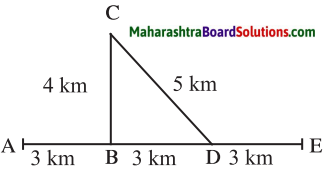Sachin and Sarneer started on a motorbike from place A, took the turn at 13, did a task at C, travelled by the route CD to D and then went on to E. Altogether, they took one hour for this journey. Find out the actual distance traversed by them and the displacement from A to E. From this, deduce their speed. What was their velocity from A to E in the direction AE’? Can this velocity be called average velocity?

Question a.
Observe the figure and answer the questionsSachin and Sarneer started on a motorbike from place A, took the turn at 13, did a task at C, travelled by the route CD to D and then went on to E. Altogether, they took one hour for this journey. Find out the actual distance traversed by them and the displacement from A to E. From this, deduce their speed. What was their velocity from A to E in the direction AE’? Can this velocity be called average velocity?
1. Actual distance = AB⎯⎯⎯⎯⎯⎯⎯⎯ + BC⎯⎯⎯⎯⎯⎯⎯⎯ + CD⎯⎯⎯⎯⎯⎯⎯⎯ + DE⎯⎯⎯⎯⎯⎯⎯⎯ = 3 + 4 + 5 + 3
Actual distance = 15 km

2. Displacement = AB⎯⎯⎯⎯⎯⎯⎯⎯ + BD⎯⎯⎯⎯⎯⎯⎯⎯ + DE⎯⎯⎯⎯⎯⎯⎯⎯
= 3 + 3 + 3
Displacement = 9 km

3. Speed =  Distance travelled  Total time
Distance = 15 km = 15 × 1000 = 15000 m
Time = 1 hr = 1 × 60 × 60 = 3600 sec.
s = 150003600 or s = 15 km1 hour  = 15km/hour
= 4.16 m/sec. or 15 km/hour

4. Velocity =  Distance travelled  Total time
Displacement = 9 km = 9 × 1000 = 9000 m
Time = 1 hr = 1 × 60 × 60 = 3600 sec
V = 90003600 or V = 9 km1 hour  = 9 km/hour
= 2.5 m/sec. or 9 km/hour

5. Yes, this velocity can be called as average velocity.

3. From the groups B and C, choose the proper words, for each of the words in group A.

Question a.
From the groups B and C, choose the proper words, for each of the words in group A.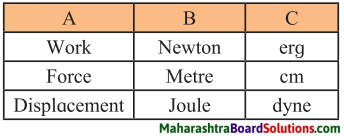Group ‘A’ Group B’ Group ‘C’ Work Joule erg Force Newton dyne Displacement Metre cm

4. A bird sitting on a wire, flies, circles around and comes back to its perch. Explain the total distance it traversed during its flight and its eventual displacement.

Question a.
The total distance the bird has traversed is the length of the distance covered by circling, but the eventual displacement are the bird is zero as its initial and final position are one and the same.

5. Explain the following concepts in your own words with everyday examples: force, work, displacement, velocity, acceleration, distance.

Question a.
Explain the following concepts in your own words with everyday examples: force, work, displacement, velocity, acceleration, distance.
1. Force: The interaction that brings about the acceleration is called force.
e.g: An ox is pulling a cart, applying brakes to a bicycle, lifting heavy iron object with a crane.

2. Work: When an object is displaced by applying a force on it, work is said to be done.
e.g: A bucketful of water is to be drawn from a well and taken to the home by walking from well to home.

3. Displacement: The minimum distance
traversed by a moving body in one direction from the original point to reach the final point is called displacement.
e.g: A rolling of a ball from point A to point B in the same direction.

4. Velocity: Velocity is the distance traversed by a body in a specific direction in unit time.
e.g: A truck is covering a distance of 40km from A to D in a straight line in 1 hour.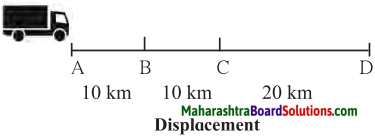5. Acceleration: It is change in velocity per second. It can be deduced.
Acceleration =  Change in velocity  Time taken for change
e.g: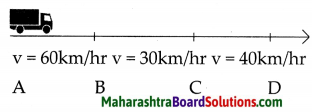(i) In the above example a truck covered the distance AB at velocity of 60 km/hr, BC at 30 km/hr and CD at 40 km/hr. (ii) It means that the velocity for the distance CD is greater than the velocity for the distance BC. (iii) From the number of seconds required for this change in velocity to take place, the change in velocity per second can be deduced. This is called acceleration (iv) Distance: The length of the route actually traversed by a moving body irrespective of the direction is called distance.
e.g: Ranjit travelled 1km. from his home to school.

6. A ball is rolling from A to D on a flat and smooth surface. Its speed is 2 cm/s. On reaching B, it was pushed continuously up to C. On reaching D from C, its speed had become 4 cm/s. It took 2 seconds for it to go from B to C. What is the acceleration of the ball as it goes from B to C.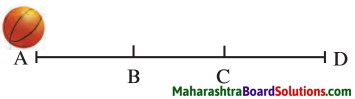Question a.
A ball is rolling from A to D on a flat and smooth surface. Its speed is 2 cm/s. On reaching B, it was pushed continuously up to C. On reaching D from C, its speed had become 4 cm/s. It took 2 seconds for it to go from B to C. What is the acceleration of the ball as it goes from B to C.As its initial and final positions are one and the same.
Initial Velocity = 2 cm/s.
Final Velocity = 4 cm/s
Time taken for the change in velocity from B to
D = 4 cm/s – 2 cm/s = 2 cm/s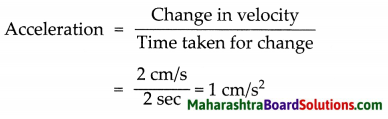7. Solve the following problems.

Question a.
A force of 1000 N was applied to stop a car that was moving with a constant velocity. The car stopped after moving through 10m. How much is the work done?
Force (F) = 1000 N
displacement (s) = 10m
work done (W) = ?
W = Fs
= 1000 × 10
W = 10,000 Joule

Question b.
A cart with mass 20 kg went 50 m in a straight line on a plain and smooth road when a force of 2 N was applied to it. How much work was done by the force?
Force (F) = 2 N
Displacement (s) = 50 m
Work done (W) = ?
W = Fs
= 2 × 50
W = 100 Joule

Project:

Question a.
Collect information about the study made by Sir Isaac Newton regarding force and acceleration and discuss it with your teacher.

Class 7 Science Chapter 7 Motion, Force and Work Important Questions and Answers

Fill in blanks:

Question 1.
Displacement is a …………. quantity.
vector

Question 2.
The …………. of an object can change even while it is moving along a straight line.
velocity

Question 3.
The …………. velocity can be different at different times.
instantaneous

Question 4.
Change in velocity per second is called …………. .
acceleration

Question 5.
The interaction that brings about the acceleration is called …………. .
force

Question 6.
The scientist …………. was the first to study force and the resulting acceleration.
Sir Isaac Nezvton

Question 7.
Ability to do work is called …………. .
Energy

Question 8.
W = …………. × S.
F

Question 9.
Unit of work is …………. and …………. .
Joule, erg

Question 10.
Unit of force is …………. and …………. .
Newton, dyne

Question 11.
Force is a …………. quantity.
vector

Question 12.
The velocity at a particular time is called …………. velocity.
instantaneous

Question 13.
The …………. of a body is the distance traversed per unit time.
speed

Question 14.
Unit of acceleration is …………. and …………. .
m/s2 and cm/s2

Question 15.
Force is measured by the …………. that it produces.
acceleration

Question 16.
Work done by a body with no displacement will be …………. .
zero

Say whether True or False, correct the false 1 statements:

Question 1.
Velocity is distance travelled per unit of time.
False. Speed is distance travelled per unit of time

Question 2.
In displacement, both distance and direction are taken into account.
True

Question 3.
Speed = Distance/time.
True

Question 4.
Change in speed per second is acceleration.
False. Change in velocity per second is acceleration

Question 5.
Work done depends on the force and the displacement.
True

Question 6.
C.G.S. unit of acceleration is m/s2.
False. C.G.S. unit of acceleration is cm/s2.

Question 7.
M.K.S. unit of force is dyne.
False. M.K.S. unit of force is Newton

Question 8.
Force is measured by the acceleration that it produces.
True

Write the difference between the following:

Question 1.
Speed and Velocity

 Speed Velocity 1. Speed is distance travelled per unit of time. 1. Velocity is the distance traversed by a body in a specific direction in unit time. 2. It is a scalar quantity. 2. It is a vector quantity. 3. Formula:Speed =  Distance traversed  Total time 3. Formula:Velocity =  Displacement  Total time

Question 2.
Distance and Displacement

 Distance Displacement 1. The length of the route actually traversed by a moving body, irrespective of the direction is called distance. 1. The minimum distance traversed by a moving body in one direction from the original point to reach the final point is called displacement. 2. It is a scalar quantity. 2. It is a vector quantity.

Solve the following problems!

Question 1.
A bus travelled 200 km in the first 3 hours and then 100 kms for the next one and a half hours and then 120 kms for the next one and a half hours. What is the average velocity of the bus if it has moved in a straight line for the whole journey.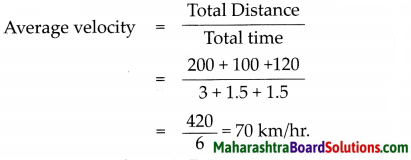Question 2.
See the diagram and calculate the Distance and Displacement travelled by the body from A to I.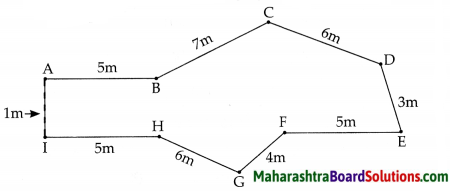Distance travelled =
A → B → C → D → E → F → G → H + I
= 5 + 7 + 6 + 3 + 5 + 4 + 6 + 5
= 41 m
Displacement = A → I in a straight line shortest distance
= 1m

Question 1.
The unit of acceleration is m/s2, verify this.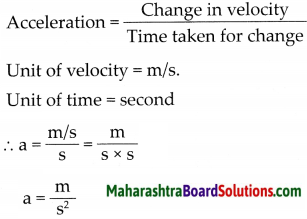Question 2.
Acceleration is a vector quantity. Is force a vector quantity too?
Yes, acceleration and force both are vector quantities, because both can be expressed completely only when magnitude and direction are given and the quantity which needs direction and magnitude both is called a vector quantity.

## निष्कर्ष

आम्हाला आशा आहे की महाराष्ट्रावरील हा लेख तुम्हाला 'सातवी विज्ञान गती, बल आणि कार्य स्वाध्याय' मदत करेल. तुम्हाला काही प्रश्न असल्यास, त्यांना खाली टिप्पणी विभागात मोकळ्या मनाने पोस्ट करा. आम्ही लवकरात लवकर तुमच्याकडे परत येऊ.
Share: